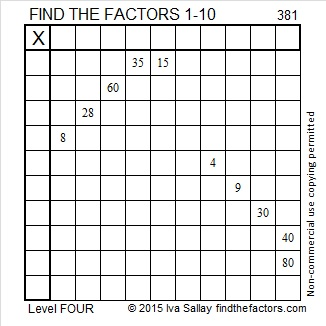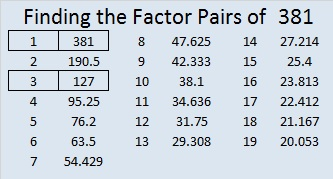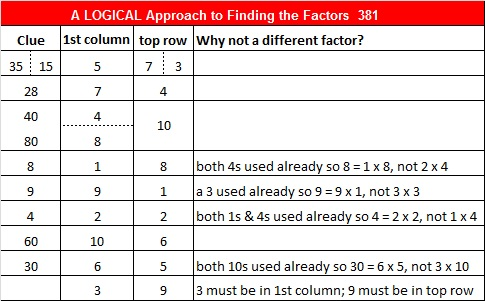# 381 and Level 4

Is 381 Prime or composite? Its digits can be grouped into 3 and 81. Both groups are divisible by 3 so 381 is composite. Scroll down past the puzzle to view its factors.Print the puzzles or type the factors on this excel file: 10 Factors 2015-02-02

• 381 is a composite number.
• Prime factorization: 381 = 3 x 127
• The exponents in the prime factorization are 1 and 1. Adding one to each and multiplying we get (1 + 1)(1 + 1) = 2 x 2 = 4. Therefore 381 has exactly 4 factors.
• Factors of 381: 1, 3, 127, 381
• Factor pairs: 381 = 1 x 381 or 3 x 127
• 381 has no square factors that allow its square root to be simplified. √381 ≈ 19.519Pythagorean triples:

• 381 – 508 – 635 which is 127 times (3 – 4 – 5 )
• 381 – 24192 – 24195 which is 3 times (127 – 8064 – 8065)
• 381 – 8060 – 8069, a primitive
• 381 – 72580 – 72581, a primitive